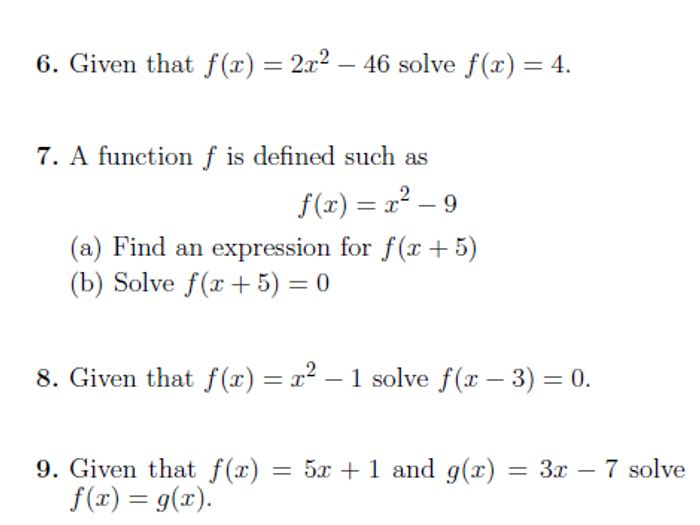9 GCSE/IGCSE Math worksheets on the following topics: percentage increase and decrease, percentages, fractions and decimals, reverse percentage, functions, composite functions, domain of a function, inverse functions, simplifying algebraic fractions, equations with algebraic fractions. Detailed solutions are provided. From https://placeformath.blogspot.com.cy/p/worksheet-shop.html

£3.32

£3.90);

(15% off)

Save for later